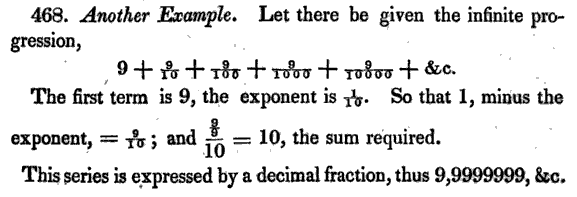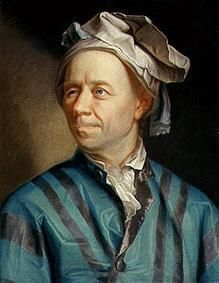### The Number 0.999...

October 14, 2010

The recent content of this blog has been somewhat heavy reading, Nobel Laureates, general relativity, and all that, so it's time for some light diversion. Mathematics has its lighter and more accessible moments, although a perusal of recent papers on arXiv might convince you otherwise. Maurice Allais, an able practitioner of applied mathematics whom I eulogized in yesterday's article, had the following opinion of mathematics:
"Any author who uses mathematics should always express in ordinary language the meaning of the assumptions he admits, as well as the significance of the results obtained. The more abstract his theory, the more imperative this obligation. In fact, mathematics are and can only be a tool to explore reality. In this exploration, mathematics do not constitute an end in itself, they are and can only be a means."

Now, the promised diversion.

So, you really think that the number "0.999..." is smaller than one? "0.999..." appears to be a real number, not a rational number, like 1/3, so how could it possibly be equal to the rational number 1? There's a 28 page paper in the The Montana Mathematics Enthusiast that reviews proofs that "0.999..." is identical to one. I discussed this number in a previous article (A Not So Limiting Case, November 8, 2007), where I presented the following proof:
• Let a = 0.999...
• then 10a = 9.999...
• subtracting 10a - a = 9.999... - 0.999
• 9a = 9
• a = 1
Here's another proof.
• 1/9 = 0.111...
• 9 x (1/9) = 0.999...
• 1 = 0.999...
Another proof, presented by Leonhard Euler in his Elements of Algebra, appears below. Euler's equation is for 9.999..., which resolves to 10, but the idea is the same.Section 468 of Euler's Elements of Algebra (John Farrar, trans, Hilliard, Gray & Co., Boston, 1836).

The principle behind Euler's proof is that any convergent infinite series of the form
a(r)1 + a(r)2 + a(r)3 + a(r)4 + ...
has the sum (ar)/(1-r), provided that |a| < 1. In our case, using a = 9 and r = (1/10) gives the decimal representation for 0.999..., and the sum 0.9/0.9 = 1.

Many students encounter this identity with disbelief. After all, even after writing many nines after the decimal point, the number still seems to fall short of one by a known amount that can be expressed exactly. That's why mathematics is both wonderful and intimidating at the same time.Leonhard Euler.### References:

Linked Keywords: Nobel Laureates; general relativity; mathematics; arXiv; Maurice Allais; The Montana Mathematics Enthusiast; Leonhard Euler; convergent infinite series.